×#### Thank you for registering.

One of our academic counsellors will contact you within 1 working day.

Click to Chat

1800-1023-196

+91-120-4616500

CART 0

• 0

MY CART (5)

Use Coupon: CART20 and get 20% off on all online Study Material

ITEM
DETAILS
MRP
DISCOUNT
FINAL PRICE
Total Price: Rs.

There are no items in this cart.
Continue Shopping• Complete JEE Main/Advanced Course and Test Series
• OFFERED PRICE: Rs. 15,900
• View Details

```Chapter 3: Pair of Linear Equations in Two Variables Exercise – 3.6

Question: 1

5 pens and 6 pencils together cost Rs 9 and 3 pens and 2 pencils cost Rs 5. Find the cost of 1 pen and 1 pencil.

Solution:

Let the cost of a pen be Rs x and that of a pencil be Rs y. Then,

5x + 6y = 9       …(i)

3x + 2y = 5       …(ii)

Multiplying equation(i) by 2 and equation (ii) by 6, we get

10x + 12y = 18             (iii)

18x + 12y = 30             (iv)

Subtracting equation(iii) by equation(iv), we get

18x - 10x + 12y - 12y = 30 – 18

8x = 12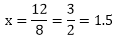Substituting x =1.5 in equation(i), we get

5 × 1.5 + 6y = 9

7.5 + 6y = 9

6y = 1.5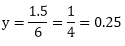Hence, cost of one pen = Rs. 1.50 and cost of one pencil = Rs. 0.25

Question: 2

7 audio cassettes and 3 videocassettes cost Rs. 1110, while 5 audio cassettes and 4 videocassettes cost Rs. 1350. Find the cost of audio cassettes and a video cassette.

Solution:

Let the cost of a audio cassette be Rs. X and that of a video cassette be Rs. y. Then,

7x + 3y = 1110             …(i)

5x + 4y = 1350             …(ii)

Multiplying equation(i) by 4 and equation(ii) by 3, we get

28x + 12y = 4440         …(iii)

15x + 4y = 4050           …(iv)

Subtracting equation (iv) from equation(iii) we get

28x - 13x + 12y - 12y = 4440 - 4050

13x = 390Substituting x = 30 in equation (i), we get

7 × 30 + 3y = 1110

210 + 3y = 1110

3y = 1110 - 210

3y = 900

y = 9003 = 300

Hence, cost of one audio cassette = Rs. 30 and cost of one video cassette = Rs. 300

Question: 3

Reena has pens and pencils which together are 40 in number. If she has 5 more pencils and 5 fewer pens, then the number of pencils would become 4 times the number of pens. Find the original number of pens and pencils.

Solution:

Let the number of pens be x and that of pencil be y, then,

x + y = 40             …(i)

and (y + 5) = 4((x - 5)

y + 5 = 4x - 20

5 + 20 = 4x - y

4x - y = 25           …(ii)

Adding equation (i) and equation(ii), we get

x + 4x = 40 + 25

5x = 65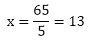Putting x = 13 in equation (i), we get

13 + y = 40

y = 40 - 13 - 27

Hence, Reena has 13 pens 27 pencils.

Question: 4

4 tables and 3 chairs, together, cost Rs. 2250 and 3 tables and 4 chairs cost Rs. 1950. Find the cost of 2 chairs and 1 table.

Solution:

Let the cost of 1 table is Rs. x and cost of 1 chair is Rs. y.
Then, by the given question,
4x + 3y = 2250 ———— (1)
3x + 4y = 1950 ———— (2)
Multiplying (1) with 3 and (2) with 4 we get,
12x + 9y = 6750 ———– (3)
12x + 16y = 7800 ———- (4)
Now subtracting (4) from (3) we get,
-7y = -1050
or, y = 150
Then, x = (1950 - 4 × 150)/3 = (1950 - 600)/3 = 1350/3 = 450
∴, The total cost of 2 chairs and 1 table is:
2y + x = 2 × 150 + 450 = 300 + 450 = 750

Question: 5

3 bags and 4 pens together cost Rs 257 whereas 4 bags and 3 pens together cost Rs. 324. Find the total cost of 1 bag and 10 pens.

Solution:

Let the cost of a bag be Rs. x and that of a pen be Rs. y. Then,

3x + 4y = 257       … (i)

and, 4x + 3y = 324           … (ii)

Multiplying equation (i) by 3 and equation (ii) by 4, we get

9x + 12y = 770     … (iii)

16x + 12y = 1296             … (iv)

Subtracting equation (iii) by equation (iv), we get

16x - 9x = 1296 - 771

7x = 525Cost of a pen = Rs. 75

Putting x = 75 in equation (i), we get

3 × 75 + 4y = 257

225 + 4y = 257

4y = 257-225

4y = 32Therefore, Cost of a pen = Rs. 8

Therefore, Cost of 10 pens = 8 × 10 = Rs. 80

Hence, the total cost of 1 bag and 10 pens = 75 + 80 = Rs. 155.

Question: 6

5 books and 7 pens together cost Rs. 79 whereas 7 books and 5 pens together cost Rs. 77. Find the total cost of 1 book and 2 pens.

Solution:

Let the cost of a book be Rs. x and that of a pen be Rs. y .Then,

5x + 7y = 79         … (i)

and, 7x + 5y = 77         … (ii)

Multiplying equation (i) by 5 and equation (ii) by 7, we get

25 + 35y = 395    … (iii)

49x + 35y = 539     … (iv)

Subtracting equation (iii) by equation (iv), we get

49x - 25x = 539 - 395

24x = 144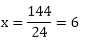Therefore, cost of a book = Rs. 6

Putting x = 6 in equation (i), we get

5 × 6 + 7y = 79

30 + 7y = 79

7y = 79 - 30

7y = 49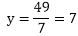Therefore, cost of a pen = Rs. 7

Therefore, cost of 2 pens = 2 × 7 = Rs. 14

Hence, the total cost of 1 book and 2 pens = 6 + 14 = Rs. 20

Question: 7

Jamila sold a table and a chair for Rs 1050, thereby making a profit of 10% on the table and 25% on the chair. If she had taken a profit of 25% on the table and 10% on the chair she would have got Rs 1065. Find the cost price of each.

Solution:

Let the cost price of the table be Rs x and the cost price of the chair be Rs y.

The selling price of the table, when it is sold at a profit of 10% = Rs x + 10x/100 = 110x/100

The selling price of the chair when it is sold at a profit of 25% = Rs y + 25y/100 = 125y/100

So, 110x/100 + 125y/100 = 1050    … (1)

When the table is sold at a profit of 25%, its selling price = Rs (x + 25x/100) = Rs 125x/100

When the chair is sold at a profit of 10%, its selling price = Rs (y + 10y/100) = Rs 110y/100

So, 125x/100 + 110y/100 = 1065   … (2)

Solve (1) and (2), to get x = 500 and y = 400

Hence, the cost price of the table is Rs 500 and the cost price of the chair is Rs 400.

Question: 8

Susan invested a certain amount of money in two schemes A and B, which offer interest at the rate of 8% and 9% per annum respectively. She received Rs 1860 as annual interest. However, if she had interchanged the amounts of investment in the two schemes, she would have received Rs 20 more as annual interest. How much money did she invest in each scheme?

Solution:

Let Susan invest Rs x in scheme A at the rate 8% per annum

And Rs y in scheme B at the rate 9% per annum

According to the given conditions,

8x + 9y = 1860     … (i)

9x + 8y = 1860 + 20           … (ii)

Multiplying (i) by 9 and by 8, we get

72x + 81y = 16740           … (iii)

72x + 64y = 15040           … (iv)

Subtracting (iv) from (iii)

Therefore, 17y = 1700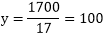y = 100

Substituting the value of y in (i), we get

8x + 9 × 100 = 1860

Therefore, 8x = 1860 - 900

8x = 960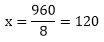x = 120

Therefore, Susan invested Rs.120 in the scheme A and Rs.100 in the scheme B.

Question: 9

The coach of a cricket team buys 7 bats and 6 balls for Rs 3800. Later, she buys 3 bats and 5 balls for Rs 1750. Find the cost of each bat and each ball.

Solution:

Let cost of each bat =Rs x

And, Cost of each ball = Rs y

Given that coach of a cricket team buys 7 bats and 6 balls for Rs 3800.

So that 7x + 6y = 3800

6y = 3800 - 7x

Divide by 6 we get

y = (3800 – 7x) /6         … (1)

Given that she buys 3 bats and 5 balls for Rs 1750.so that

3x + 5y = 1750

Plug the value of y

3x + 5 ((3800 – 7x)/6) = 1750

Multiply by 6 we get

18x + 19000 – 35x = 10500

-17x = 10500 – 19000

-17x = -8500

x = – 8500/– 17

x = 500

Put this value in equation first we get

y = (3800 – 7 * 500) / 6

y = 300/6

y = 50

Hence cost of each bat = Rs 500 and cost of each balls is Rs 50

Question: 10

A lending library has a fixed charge for the first three days and an additional charge for each day thereafter. Saritha paid Rs 27 for a book kept for seven days, while Susy paid Rs 21 for the book she kept for five days. Find the fixed charge and the charge for each extra day.

Solution:

Let the fixed charge for first three days and each day charge thereafter be Rs x and Rs y respectively.
According to the question,
x + 4y = 27 … (i)
x + 2y = 21 … (ii)
Subtracting equation (ii) from equation (i), we get
2y = 6
y = 3 … (iii)
Putting in equation (i), we get
x + 12 =27
x = 15
Hence, fixed charge = Rs 15 and Charge per day = Rs 3.

Question: 11

The cost of 4 pens and 4 pencil boxes is Rs 100. Three times the cost of a pen is Rs 15 more than the cost of a pencil box. Form the pair of linear equations for the above situation. Find the cost of a pen and a pencil box.

Solution:

Let price of pens be = Rs. x
let price of pencil boxes = Rs. y
4x + 4y = 100   …..1
3x -  y = 15     …... 2
so using (1) and (2)

4x + 4y = 100

4{3x – y = 15}

So equation becomes

4x + 4y = 100

12x - 4y = 60

It becomes

16x = 160

x =  Rs.10

Substituting this value in (1)

40 + 4y = 100

So, y = Rs. 15

Question: 12

One says, “Give me a hundred, friend! I shall be twice as rich as you.” The other replies, “If you give me ten, I shall be six times as rich as you,”. Tell me the amount of their respective capital.

Solution:

Suppose two friends were having Rs x and y with them.
as per condition  given in the question, we obtain
x + 100 = 2(y − 100)
x + 100 = 2y − 200
x − 2y = −300 ... (i)
and  6(x − 10) = (y + 10)
6x − 60 = y + 10
6x − y = 70 ... (ii)
Multiplying equation (ii) by 2, we obtain
12x − 2y = 140 ... (iii)
Subtracting equation (i) from equation (iii), we obtain
11x = 140 + 300
11x = 440
x = 40
Using this in equation (i), we obtain
40 − 2y = −300
40 + 300 = 2y
2y = 340
y = 170
Therefore, those friends had Rs 40 and Rs 170 with them respectively.

Question: 13

A and B have a certain number of mangoes. A says to B “If you give 30 of your mangoes I will have twice as many left with you.” B replies, ” If you give me 10, I will have thrice as many as left with you. How many mangoes does each have?

Solution:

Assume A has x mangoes and B has y mangoes.
(x + 30) = 2(y - 30) …….. (Eq. 1)
à x = 2y - 90
3(x - 10) = (y + 10) …….. (Eq. 2)

à 3x = y + 40
à 3(2y - 90) = y + 40 (using x from Eq. 1)
à 6y - y = 310
à y = 62
So, x = 124 - 90 = 34
Answer: A has 34 mangoes and B has 62 mangoes.

Question: 14

Vijay had some bananas and he divided them into 2 lots A and B. He sold the first lot Rs 2 for 3 bananas and the 2nd lot Rs 1 per banana and got a total of Rs 400. If he had sold the first lot Rs 1 per banana and the 2nd lot Rs 4 for 5 bananas, his total collection would have been Rs 460. Find the total no. of bananas he had?

Solution:

Let the number of bananas in lot A be x and in lot B be y.

Condition I:

In lot A, cost of 3 bananas = Rs. 2

Therefore, In lot A, cost of x bananas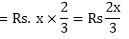In lot B, cost of 1 banana = Re. 1

Therefore, In lot B, cost of y bananas = Rs. y

Total cost of lot A and lot B = Rs. 400

Therefore, 2x/3 + y = 400

Therefore, 2x + 3y = 1200    … (1)

Condition II:

In lot A, cost of 1 banana = Rs. 1

Therefore, In lot A, cost of x bananas = Rs. x

In lot B, cost of 5 bananas = Rs. 4

Therefore, In lot B, cost of y bananas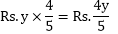Total cost of lot A and lot B = Rs. 460Therefore, 5x + 4y = 2300  … (2)

Multiplying equation (1) by 5, we get

10x + 15 y = 6000         … (3)

Multiplying equation (2) by 2, we get

10x + 8y = 4600           … (4)

Subtracting eq. (3) from eq. (4)

We get, – 7y = –1400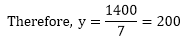Substituting the value of y in (1), we get

2x + 3 × 200 = 1200

Therefore, 2x = 1200 – 600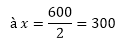Number of bananas in lot A = 300

Number of bananas in lot B = 200

Therefore, total number of bananas = 300 + 200 = 500

Question: 15

On selling a T.V. at 5% gain and a fridge at 10% gain shop keeper gain Rs. 2000 but if he sells the T.V. at 10% gain in the fridge at 5% loss. He gains Rs. 1500 on the transaction. Find the actual price of T.V. and fridge.

Solution:

To solve these questions, you can form simultaneous equations:
First let price of T.V. be x  and that of the fridge be y
5% gain on chair = 5/100x = 0.05x
10% gain on fridge = 10/100y = 0.1y
Therefore 0.05x + 0.1 y = 2000(total profit)…..first equation(hence 5x + 10y = 200000)

10% gain on T.V. = 10/100x = 0.1x
5% loss on fridge = -5/100y = – 0.05y

Therefore 0.1x – 0.05y = 1500……our second equation (hence, 5y = 10x - 150000…y = (10x-150000)/5….y = 2x - 30000
solve by substitution: y = 2x - 30000
5x + 10y = 200000
5x + 10(2x - 30000) = 200000
5x + 20x - 300000 = 200000
25x = 500000
x = 20000
y = 2x - 30000
= 2(20000) - 30000 = 40000 - 30000
= 10000

Therefore, the price of the T.V. = Rs. 20,000
price of the fridge = Rs. 10,000
```### Course Features

• 728 Video Lectures
• Revision Notes
• Previous Year Papers
• Mind Map
• Study Planner
• NCERT Solutions
• Discussion Forum
• Test paper with Video Solution